## 7th Class Maths Concept

Studying maths in VII class successfully means that children take responsibility for their learning and learn to apply the concepts to solve problems.

### 1. INTEGERS

Natural numbers: All the counting numbers starting from 1 are called Natural numbers.

1, 2, 3… Etc.

Whole numbers: Whole numbers are the collection of natural numbers including zero.

0, 1, 2, 3 …

Integers: integers are the collection of whole numbers and negative numbers.

….,-3, -2, -1, 0, 1, 2, 3,…..

Integers on a number line:Operations on integers:

3 + 4 = 7-2 + 4 = 2Subtraction of integers on a number line:-

6 – 3 = 3Multiplication of integers on a number line:-

2 × 3 ( 2 times of 3) = 63 × (- 4 ) ( 3 times of -4) = -12Multiplication of two negative integers:

• To multiply two negative integers, first, we multiply them as whole numbers and put plus sign before the result.
• The multiplication of two negative integers is always negative.

Ex:- -3 × -2 = 6,  -10 × -2 = 20 and so on.

Multiplication of more than two negative integers:

• If we multiply three negative integers, then the result will be a negative integer.

Ex:- -3 ×   -4 ×   -5 = -60,  -1× -7 × -4 = -28 and so on.

• If we multiply four negative integers, then the result will be a positive integer.

Ex:- -3 ×   -4 ×  -5 × -2  = 120,  -1× -7 × -4  × -2 = 56 and so on.

Note:-

1. If the no. of negative integers is even, then the result will be positive.

2. If the no. of negative integers is odd, then the result will be negative.Division of integers:

• The division is the inverse of multiplication.
• When we divide a negative integer by a positive integer or a positive integer by a negative integer, we divide them as whole numbers then put negative signs for the quotient.

Ex:- -3 ÷ 1 = 3, 4 ÷ -2 = -2 and so on.

When we divide a negative integer by a negative integer, we get a positive number as the quotient.

Ex:- -3 ÷ -1 = 3, -4 ÷ -2 = 2 and so on.

#### Properties of integers:

1.Closure property:-2.commutative property:-3.associative property:-1 + 0 = 0 + 1 = 1,   10 + 0 = 0 + 10 = 10

•For any integer ‘a’, a + 0 = 0 + a

2 + (-2) = (-2) + 2 = 0,  5 + (-5) = (-5) + 5 = 0

•For any integer ‘a’, a+ (-a) = (-a) + a = 0

•Additive inverse of a = -a and additive inverse of (-a) = a

Multiplicative identity:-

2 × 1 = 1 × 2 = 2,    5 × 1 = 1 × 5 = 5

•For any integer ‘a’, a × 1 = 1 × a = a

•1 is the multiplicative identity.

multiplicative inverse:-

For any integer ‘a’, 1/a × a = a × 1/a = 1

• multiplicative inverse of a = 1/a
• Multiplicative inverse of  1/a = a.

distributive property:-

For any three integers a, b and c,    a × (b + c) = (a × b) + (a × c).

3 × (2 + 4) = 18

(3 × 2) + (3 × 4) = 6 + 12 = 18

∴ 3 × (2 + 4) = (3 × 2) + (3 × 4).

### 2. FRACTIONS, DECIMALS AND RATIONAL NUMBERS

Fraction: A fraction is a number that represents a part of the whole. A group of objects is divided into equal parts, then each part is called a fraction.

The proper and improper fractions:

In a proper fraction, the numerator is less than the denominator.

Ex: – 1/5, 2/3, and so on.

In an improper fraction, the numerator is greater than the denominator.

Ex: – 5/2,11/5 and so on.

Comparing fractions:

Like fractions: – We have to compare the like fractions with the numerator only because the like fractions have the same denominator. The fraction with the greater numerator is greater and the fraction with the smaller numerator is smaller.

Unlike fractions: –

With the same numerator: For comparing unlike fractions, we have to compare denominators when the numerator is the same. The fraction with a greater denominator is smaller and the fraction with a smaller denominator is smaller.

Note: – To find the equivalent fractions of both the fractions with the same denominator, we have to take the LCM of their denominators.

∗ Like Fractions:∗ Unlike fractions:Subtraction of fractions:

∗ Like fractions: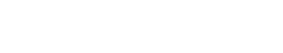Unlike fractions: – First, we have to find the equivalent fraction of given fractions and then subtract them as like fractions

Multiplication of fractions:

Multiplication of fraction by a whole number: –

Multiplication of numbers means adding repeatedly.• To multiply a whole number with a proper or improper fraction, we multiply the whole number with the numerator of the fraction, keeping the denominator the same.

2.Multiplication of fraction with a fraction: –Division of fractions:

⇒ 6 one-thirds in two wholes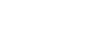Note:

• dividing by a fraction is equal to multiplying the number by its reciprocal.
• For dividing a number by mixed fraction, first, convert the mixed fraction into an improper fraction and then solve it.

1.Division of a whole number by a fraction: –2.Division of a fraction by another fraction: –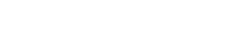Decimal number or fractional decimal:

In a decimal number, a dot(.) or a decimal point separates the whole part of the number from the fractional part.

The part right side of the decimal point is called the decimal part of the number as it represents a part of 1. The part left to the decimal point is called the integral part of the number.

Note: –

• while adding or subtracting decimal numbers, the digits in the same places must be added or subtracted.
• While writing the numbers one below the other, the decimal points must become one below the other. Decimal places are made equal by placing zeroes on the right side of the decimal numbers.
###### Comparison of decimal numbers:

while comparing decimal numbers, first we compare the integral parts. If the integral parts are the same, then compare the decimal part.

Ex: – which is bigger: 13.5 or 14.5

Ans: 14.5

Which is bigger: 13.53 or 13. 25

Ans: 13.53

Multiplication of decimal numbers: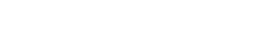Multiplication of decimal numbers by 10, 100, and 1000: –Here, we notice that the decimal point in the product shifts to the right side by as many zeroes as in 10, 100, and 1000.

Division of decimal number:

Division of decimal number by 10,100 and 1000: –Here, we notice that the decimal point in the product shifts to the left side by as many zeroes as in 10, 100, and 1000.

Rational numbers:

The numbers which are written in the form of p/q, where p, q are integers, and q ≠ 0, are called rational numbers.

Rational numbers are a bigger collection of integers, negative fractional numbers, positive fractional numbers.

Ex: – 1, 2, -1/2, 0 etc.

### 3. SIMPLE EQUATIONS

Equation: Equation is the condition of a variable. It says that two expressions are equal.

• An equation has two sides LHS and RHS, on both sides of the equality of sign.
• One of the expressions of the equation is must have a variable.
• If we interchange the expressions from LHS to RHS, the equation remains the same

Ex: – x + 2 = 5; 2 = x + 3

Balanced equation:

In an equation, if LHS =RHS, then that equation is balanced.

If the same number is added or subtracted on both sides of the balanced equation, the equation remains will the same.

Ex: 8 + 3 = 11

If add 2 on both sides ⇒ LHS = 8 + 3 + 2 = 13

RHS = 11 + 2 = 13

∴ LHS = RHS

8 +3 = 11 if subtract 2 on both sides

LHS = 8 + 3 – 2 = 9

RHS = 11 – 2 = 9

∴ LHS = RHS###### Using algebraic equations in solving day to day problems:
2. Denote the unknown or quantity to be found with some letters such as x, y, z …etc.
3. Write the problem in the form of an algebraic equation by making a relation among the quantities.
4. Solve the equation.
5. Check the solution

### 4. LINES AND ANGLES

Complimentary angles: When the sum of the angles is 900, the angles are called complementary angles.

Ex: 300, 600; 200, 700Supplementary angles: When the sum of the angles is 1800, the angles are called  Supplementary angles.

Ex: 1200, 600; 1100, 700 and soon.Adjacent angles: The angle having a common Arm and a common vertex are called Adjacent angles.⇒ ∠AOC and ∠BOC adjacent angles.

Vertically opposite angle: If two lines are intersecting at a point, then the angles that are formed opposite to each other at that point are called vertically opposite angles.Transversal: A line that intersects two or more lines at distinct points is called a transversal.#### Angles made by a transversal:

Corresponding angles:

Two angles that lie on the same side of the transversal and one interior and another one exterior are called corresponding angles.

Alternate angles:

Two angles which are the lies opposite side of the transversal and both interior or exterior are called Alternate angles.

∠1, ∠7; ∠2, ∠8 are exterior alternate angles

∠3, ∠5; ∠4, ∠6 are interior alternate angles.

∠3, ∠6; ∠4, ∠5 interior angles same side of the transversal.

Transversal on parallel lines:

If pair of parallel lines are intersected by a transversal then the angles of each pair of corresponding angles are equal

•If pair of parallel lines are intersected by a transversal then the angles of each pair of interior alternate angles are equal.

∠3= ∠5; ∠4= ∠6

•If pair of parallel lines are intersected by a transversal then the angles of each pair of exterior alternate angles are equal.

∠1= ∠7; ∠2= ∠8

•If pair of parallel lines are intersected by a transversal then the angles of each pair of interior angles on the same side of the transversal are supplementary.

∠3+∠6= 1800; ∠4+ ∠5 = 1800

Note:

1.If a transversal intersects two lines and the pair of corresponding angles are equal, then the lines are parallel.

2.If a transversal intersects two lines and the pair of alternate angles are equal, then the lines are parallel.

3.If a transversal intersects two lines and the interior angles on the same side of the transversal are supplementary, then the lines are parallel.

### 5. TRIANGLE AND ITS PROPERTIES

Triangle:
A closed figure formed by three-line segments is called a triangle.

In ∆ABC,

Classification of triangles:

Triangles can be classified according to the properties of their sides and angles.

According to sides:

Based on sides triangles are three types:

• Scanlan triangle (ii) Isosceles Triangle (iii) equilateral triangle

According to angles:

• Acute-angled triangle (ii) Right-angled triangle (iii) Obtuse-angled triangleRelationship between the sides of a triangle:

1. The sum of the lengths of any two sides of a triangle is greater than the third side.

1. The difference between the lengths of any two sides of a triangle is less than the third side.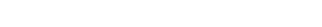The altitude of a triangle:

A perpendicular line drawn from a vertex to its opposite side of a triangle is called the Altitude of the triangle.

Median of a triangle:In a triangle, a line drawn from the vertex to the mid-point of its opposite side is called the median of the triangle.

Medians of a triangle are concurrent. We can draw three medians in a triangle.The point of concurrence of medians is called the centroid of the triangle. It is denoted by G

Angle-sum property of a triangle:
Some of the angles in a triangle is 1800

∠A + ∠B + ∠C = 1800

An exterior angle of a triangle:

When one side of the triangle is produced, the angle thus formed is called an exterior triangle.

Exterior angle property:- The exterior angle of a triangle is equal to the sum of two interior opposite angles.x0+ y0 = z0

## 6.RATIO – APPLICATIONS

Ratio: Comparison of two quantities of the same kind is called ‘Ratio.

The ratio is represented by the symbol ‘:’

If the ratio of two quantities ‘a’ and ‘b’ is a : b, then we read this as ‘a is to b’

The quantities ‘a’ and ‘b’ are called terms of the ratio.

Proportion: if two ratios are equal, then they are said to be proportional.
‘a’ is called as first term or antecedent and ‘b’ is called a second term or consequent.

If a: b = c : d, then a, b, c, d are in proportion and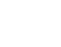⇒ ad = bc.The product of means = the product of extremes

Unitary method:  The method in which we first find the value of one unit and then the value of the required no. of units is known as the unitary method.

Direct proportion: In two quantities, when one quantity increase(decreases) the other quantity also increases(decreases) then two quantities are in direct proportion.

Percentages:

‘per cent’ means for a hundred or per every hundred. The symbol % is used to denote the percentage.

1% means 1 out of 100, 17% means 17 out of 100.

Profit and Loss:

Selling price = SP; Cost price = CP

If SP > CP, then we get profit

Profit = SP – CP

SP = CP + profit

If SP < CP, then we get a loss

Loss = CP – SP

SP = CP – Loss

Simple interest:

Principle: – The money borrowed or lent out for a certain period is called the Principle.

Interest: – The extra money, for keeping the principle paid by the borrower is called interest.

Amount: – The amount that is paid back is equal to the sum of the borrowed principal and the interest.

Amount = principle + interest

## 7.DATA HANDLING

Data: The information which is in the form of numbers or words and helps in taking decisions or drawing conclusions is called data.

Observations: The numerical entries in the data are called observations.

Arithmetic Mean: The average data is also called an Arithmetic mean.

The arithmetic mean always lies between the highest and lowest observations of the data.

When all the values of the data set are increased or decreased by a certain number, the mean also increases or decreases by the same number.

Mode: The most frequently occurring observation in data is called Mode.

If data has two modes, then it is called bimodal data.

Note: If each observation in a data is repeated an equal no. of times, then the data has no mode.

Median: The middlemost observation in data is called the Median.

Arrange given data in ascending or descending order.

If a data has an odd no. of observations, then the middle observation is the median.

If a data has even no. of observations, then the median is the average of middle observations.

Bar graph:

Bar graphs are made up of uniform width which can be drawn horizontally or vertically with equal spacing between them.

The length of each bar tells us the frequency of the particular item.

Ex:

It represents two observations side by side.

Ex:Pie chart: A circle can be divided into sectors to represent the given data

Ex:

 Budget Amount in rupees Food 1200 Education 800 Others 2000 Savings 5000 Total income 9000## 8.CONGRUENCY OF TRIANGLES

Congruent figures: Two figures are said to be congruent if they have the same shape and size.Congruency of line segments:  If two-line segments have the same length, then they are congruent. Conversely, if two-line segments are congruent, then they have the same length.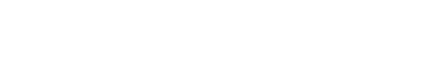Congruency of Triangles:

Two triangles are said to be congruent if (i) their corresponding angles are equal (ii) their corresponding sides are equal.
Ex: In ∆ ABC, ∆ DEF∠A ≅ ∠D; ∠B≅ ∠E; ∠C ≅ ∠F

AB ≅ DE; BC≅ EF; AC ≅ DF

∴∆ABC ≅ ∆DEF

The criterion for congruency of Triangles:

1.Side -Side -Side congruency (SSS): –
If three side of a triangle is equal to the corresponding three sides of another triangle, then the triangles are congruent.∴∆ABC ≅ ∆DEF

2.Side -Angle -Side congruency (SAS): –
If two sides and the angle included between the two sides of a triangle are equal to the corresponding two sides and the included angle of another triangle, then the triangles are congruent.∴ ∆ABC ≅ ∆DEF

3.Angle – Side -Angle congruency (ASA): –
If two angles and included side of a triangle are equal to the corresponding two angles and included side of another triangle, then the triangles are congruent.∴ ∆ABC ≅ ∆DEF

4.Right angle – Hypotenuse – Side congruence (RHS): –

If the hypotenuse and one side of a right-angled triangle are equal to the corresponding hypotenuse and side of the other right-angled triangle, then the triangles are Equal.∴∆ABC ≅ ∆DEF

## 9.CONSTRUCTION OF TRIANGLES

The no. of measurements required to construct a triangle = 3

A triangle can be drawn in any of the situations given below:

• Three sides of a triangle
• Two sides and the angle included between them.
• Two angles and the side included between them.
• The hypotenuse and one adjacent side of the right-angled triangle.

Construction of a triangle when measurements of the three sides are given:

Ex: construct a triangle ABC with sides AB = 4cm, BC = 7cm and AC = 5cm

Step of constructions:

Step -1: Draw a rough sketch of the triangle and label it with the given measurements.Step -2: Draw a line segment of BC of length 7cm.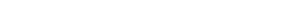Step -3: with centre B, draw an arc of radius 4cm, draw another arc from C with radius 5cm such that it intersects first at A.

Step -4: join A, B and A, C. The required triangle ABC is constructed.Construction of a triangle when two sides and the included angle given:

EX: construct a triangle ABC with sides AB = 4cm, BC = 6cm and ∠B=600

Step of constructions:

Step -1: Draw a rough sketch of the triangle and label it with the given measurements.Step -2: Draw a line segment of AB of length 4cm.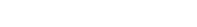Step -3: draw a ray BX making an angle 600 with AB.Step -4: draw an arc of radius 5cm from B, which cuts ray BX at C.Step -5: join C and A, we get the required ∆ABC.Construction of a triangle when two angles and the side between the angles given:

Ex: construct a triangle PQR with sides QR = 4cm, ∠Q= 1200 and ∠R= 400

Step of constructions:

Step -1: Draw a rough sketch of a triangle and label it with the given measurements.Step -2: Draw a line segment QR of length 4 cm.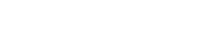Step -3: Draw a ray RX, making an angle 400 with QR.Step -4: Draw a ray QY, making an angle 1000 with QR, which intersects ray RX.

Step -5: Mark the intersecting point of the two rays as P. Required triangle PQR is constructed.Construction of a triangle when two sides and the non-included angles are given:

Ex: construct a triangle MAN with sides MN = 4cm, AM = 3cm and ∠A= 400

Step of constructions:

Step -1: Draw a rough sketch of a triangle and label it with the given measurements.Step -2: Draw a line segment MA of length 0f 5cm.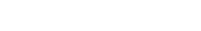Step -3: Draw a ray AX making an angle 400 with the line segment MA.

Step -4: With M as the centre and radius 3 cm draw an arc to cut ray AX. Mark the intersecting point as N.Step -5: join M, N, then we get the required triangle MAN.Construction of a right-angled triangle when hypotenuse and sides are given:

Ex: construct a triangle ABC, right angle at B and AB = 4cm, Ac = 5cm

Step of constructions:
Step -1: Draw a rough sketch of a triangle and label it with the given measurements.Step -2: Draw a line segment BC of length 0f 4cm.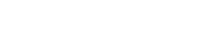Step -3: Draw a ray BX perpendicular to BC at B

Step -4: Draw an arc from C with a radius of 5cm to intersect ray BX at A.Step -5: Join A, C, then we get the required triangle ABC.## 10.ALGEBRAIC EXPRESSIONS

Variable: It is a dependent term. It takes different value.

Ex: m, x, a, etc.

Constant:  It is an independent term. It has a fixed value.

Like terms and Unlike terms: If the terms contain the same variable with the same exponents, then they are like terms otherwise, unlike terms.

Ex: 3x, –4x, x are like terms

3x, 4y, 4 are unlike terms

Coefficient: Coefficient is a term which the multiple of another term (s)

EX: In 5x. 5 is the coefficient of x and x is the coefficient of 5

5 is a numerical coefficient

x is the literal coefficient

Expression: An expression is a single term or a combination of terms connected by the symbols ‘+’ (plus) or ‘−’ (minus).

Ex: 2x – 3. 3x, 2 +3 – 4 etc.

Numerical Expressions:  If every term of an expression is constant, then the expression is called numerical expression.

Ex: 2 + 3 + 5, 2 – 4 – 7, 1 + 5 – 4 etc.

Algebraic expression:  If an expression at least one algebraic term, then the expression is called an algebraic expression.

Ex: x + y, xy, x – 3, 4x + 2 etc.

Note: Plus (+) and Minus (−) separate the terms

Multiplication (×) and Division (÷) do not separate the terms.

Types of Algebraic expressions:

Monomial: – If an expression has only one term, then it is called a monomial.

Ex: 2x2, 3y, x, y, xyz etc.

Binomial: If an expression has two unlike terms, then it is called binomial.

Ex: 2x+ 3y, x2+ y, x +yz2 etc.

Trinomial: If an expression has three unlike terms, then it is called trinomial.  Ex: 2x+ 3y + 4xy, x2+ y + z, x2 y +yz2 + xy2 etc.

Multinomial: If an expression has more than three unlike terms, then it is called multinomial.

Ex: 2x+ 3y + 4xy +5, x2+ y + z – 4y + 6 ,

x2 y +yz2 + xy2 – 4xy + 8yz etc.

Degree of a monomial: The sum of all exponents of the variables present in a monomial is called the degree of the monomial.

Ex: Degree of 5xy3

An exponent of x is 1 and an exponent of y is 3

Sum of exponents = 1 + 3 = 4

∴ degree of 5xy3 is 4

Degree of an Algebraic Expression: The highest exponent of all the terms of an expression is called the degree of an Algebraic expression.

Ex: degree of x2 + 3x + 4x3 is 3

degree of 3xy + 6x2y + 5x2y2 is 4

The sum of two or more like terms is a like term with a numerical coefficient that is equal to the sum of the numerical coefficients of all the like terms in addition.

Ex: 3x + 2x = (3 + 2) x = 5x

4x2y + x2y = (4 + 1) x2y = 5x2y

Subtraction of like terms:

The difference of two like terms is a like term with a numerical coefficient is equal to the difference between the numerical coefficients of the two like terms.

Ex: 3x − 2x = (3 − 2) x = x

4x2y −2 x2y = (4 −2) x2y = 2x2y

Note: (i) addition and subtraction are not done for unlike terms. (ii) If no terms of an expression are alike then it is said to be in the simplified form.

The standard form of an Expression:

In an expression, if the terms are in such a way that the degree of the terms is in descending order, then the expression is said to be in standard form.

Ex: 5 – 2x2 + 4x +3x3

Standard form is 3x3 – 2x2 + 4x + 5

Finding the value of an expression:

Example: find the value of expression x3 + y + 3, when x = 1 and y = 2

Sol: given expression is x3 + y + 3

Substitute x = 1 and y = 2 in above expression

(1)3 + 2 + 3 = 1 + 2 + 3 = 6

This is in two ways: (i) Column or Vertical method (ii) Row or Horizontal method.

Column or Vertical method:

Step –1: Write the expression in standard form if necessary.

Step –2: write one expression below the other such that the like terms come in the same column.

Step –3: Add the like terms column-wise and write the result just below the concerned column.

Ex: Add x2 + 3x + 5, 3 – 2x + 3x2 and 3x – 2

Row or Horizontal method.

Step –1: Write the expression in standard form if necessary.

Step –2:  Re-arrange them term by grouping the like terms.

Step –3: Simplify the coefficients.

Step –4: Write the resultant expression in standard form.

Ex: Add x2 + 3x + 5, 3 – 2x + 3x2 and 3x –2

Sol: (x2 + 3x + 5) + (3 – 2x + 3x2) + (3x –2)

= (x2 + 3x2) + (3x – 2x + 3x) + (5 + 3 – 2)

= (1 + 3) x2 + (3 – 2 + 3) x + 6

=4x2 + 4x + 6

For every algebraic expression there exist another algebraic expression such that their sum is zero. These two expressions are called the additive inverse of each other.

Subtraction of algebraic expressions:

This is in two ways: (i) Column or Vertical method (ii) Row or Horizontal method.

Column or Vertical method:

Step –1: Write the expression in standard form if necessary.

Step –2: write one expression below the other such that the expression to be subtracted comes in the second row and the like terms come one below the other.

Step –3: Change the sign of every term of the expression in the second row to get the additive inverse of the expression.

Step –4: Add the like terms column-wise and write the result just below the concerned column.

Ex: Subtract: x2 + 3x + 5 from 3x2 + 4x – 3

Sol:

Row or Horizontal method:

Step –1: Write the expressions in one row with the expression to be subtracted in a bracket with assigning a negative sign to it.

Step –2:  Add the additive inverse of the second expression to the first expression.

Step –3: Group the like terms and add or subtract.

Step –4: Write the resultant expression in standard form.

Ex: Subtract: x2 + 3x + 5 from 3x2 + 4x – 3

Sol:   3x2 + 4x – 3 – (x2 + 3x + 5)

= 3x2 + 4x – 3 – x2 – 3x – 5

= (3 – 1) x2 + (4 – 3) x + (– 3 – 5)

= 2x2 + x – 8

## 11.EXPONENTS

We know that,

a × a = a2 (a raised to the power of 2)

a × a × a = a3 (a raised to the power of 3)

a × a × a × a × a × a ×…. m times = am

am is in exponential form

a is called base, m is called exponent or index.

Laws of exponents:

Standard form:  A number that is expressed as the product of the largest integer exponent of 10 and a decimal number between 1 and 10 is said to be in standard form.

Ex: 1324 in standard form is 1.324 × 103.

Quadrilateral: A Quadrilateral is a closed figure with four sides, four angles and four vertices.• AB, BC, CD, and AD are sides.
• A, B, C and D are the vertices.
• ∠ABC, ∠BCD, ∠CDA and ∠DAC are the angles.

The line segment joining the opposite vertices of a quadrilateral are called the diagonals of the Quadrilateral. In the above figure AC, BD is the diagonals.

The two sides of a Quadrilateral that have a common vertex are called the adjacent sides of the Quadrilateral. From the above figure, AB, BC; BC, CD; CD, DA and DA, AB are the adjacent sides.

The two angles of a Quadrilateral that have a common side are called the adjacent angles of the Quadrilateral. From the above figure, ∠A, ∠B; ∠B, ∠C; ∠C, ∠D and ∠D, ∠A is the adjacent angles.

The two sides of a quadrilateral, which do not have a common vertex are called opposite sides of a quadrilateral. From the above figure, AB, CD; BC, DA are the opposite sides.

The two angles of a quadrilateral, which do not have a common side are called opposite angles of a quadrilateral. From the above figure, ∠A, ∠C; ∠B, ∠D are the opposite angles.

Interior and exterior of a Quadrilateral:In a Quadrilateral ABCD, S, N are interior points, M, P are exterior points and A, B, C, D and Q are lies on the Quadrilateral.

A Quadrilateral is said to be a convex Quadrilateral if all line segments joining points in the interior of the Quadrilateral also lie in the interior of the Quadrilateral.A Quadrilateral is said to be a concave Quadrilateral if all line segments joining points in the interior of the Quadrilateral not lie in the interior of the Quadrilateral.Angle sum property of a quadrilateral:

The Sum of the angle in a Quadrilateral is 3600In a Quadrilateral ABCD, ∠A + ∠B + ∠C + ∠D = 3600

1.Trapezium:

In a Quadrilateral, one pair of opposite sides are parallel then it is Trapezium.In a Trapezium ABCD, AB∥ DC; AC, BD are diagonals.

2.Kite:

In a Quadrilateral two distinct consecutive pairs of sides are equal in length then it is called a Kite.In a Kite ABCD, AB = BC; AD = DC AC, BD are diagonals.

3.Parallelogram:

In a Quadrilateral, two pairs of opposite sides are parallel then it is Parallelogram.In a Parallelogram ABCD, AB∥ DC, AD∥ BC; AD, BD are diagonals.

Properties of parallelogram: –

• The opposite sides of a parallelogram are equal in length.
• The opposite angles are equal in measure.
• The sum of the adjacent angles is 1800
• Diagonals are bisected to each other and not equal in length.

4.Rhombus:

In a parallelogram in which two adjacent sides are equal, then it is a Rhombus.In a Parallelogram ABCD, AB∥ DC, AD∥ BC; AD, BD are diagonals.

Properties of Rhombus: –

• All sides of a Rhombus are equal in length.
• The opposite angles are equal in measure.
• The sum of the adjacent angles is 1800
• Diagonals are bisected to each other perpendicularly and not equal in length.

5.Rectangle:

In a parallelogram all angles are equal, then it is a Rectangle.Properties of Rectangle: –

• The opposite sides are equal in length.
• Each angle is 900.
• The sum of the adjacent angles is 1800
• Diagonals are bisected to each other and not equal in length.
• Each diagonal divides the rectangle into two congruent triangles.

6.Square:

In a rectangle adjacent sides are equal, then it is a Square.Properties of Square: –

• All sides of a square are equal in length.
• Each angle is 900.
• The sum of the adjacent angles is 1800
• Diagonals are bisected to each other and equal in length.
• Each diagonal divides the square into two congruent triangles.

## 13.AREA AND PERIMETER

Area of a parallelogram:

The area of the parallelogram is equal to the product of its base (b) and the height(h)

Area of a Triangle:

The area of the triangle is equal to half the product of its base (b) and height (h).

In a Right-angled triangle, two of its sides can be the height.
Area of a Rhombus:The area of the Rhombus is equal to half the product of its diagonals

Area of rhombus = ½ d1 × d2 square units.

Circumference of the circle:Circumference of circle = 2πr = πd

Area of the rectangular path:Area of Rectangular path = area of the outer rectangle – are of the inner rectangle

## 14. UNDERSTANDING 2D AND 3D SHAPES

Net: Net is a short of skeleton-outline in 2d, which when folded the result in 3d shape.

Nets of 3D shapes:

1.Cube:

2.Cylinder:

3.Pyramid:

Oblique Sketches:

Oblique sketches are drawn on a grid paper to visualise 3D shapes.

Ex: Draw an oblique sketch of a 3×3×3 cubeStep-1: Draw the front faceStep-2: Draw the opposite face, which is the same as the front face. The sketch is somewhat offset from Step-1Step-3: Join the corresponding corners.Step-4: Redraw using dotted lines for hidden edges.Isometric Sketches:

Isometric sketches are drawn on a dot isometric paper to visualise 3D shapes.

Ex: Draw an oblique sketch of a 2×3×4 cuboidStep-1: Draw a rectangle to show the front face.Step-2:  Draw four parallel line segments of length 3cm.Step-3: Connect the corresponding corners with appropriate line segmentsStep-4: This is an isometric sketch of a cuboid## 15.SYMMETRY

Line of symmetry: The line which divides a figure into two identical parts is called the line of symmetry or axis of symmetry.An object can have one or more than one lines of symmetry.

Regular polygon:

If a polygon has equal sides and equal angles, then the polygon is called a Regular polygon.

Lines of symmetry for Regular polygons:

 Regular polygon No. of sides No. of axes of symmetry Triangle 3 3 Square 4 4 Pentagon 5 5 Polygon n n

Rotational symmetry: If we rotate a figure, about a fixed point by a certain angle and the figure looks the same as before, then the figure has rotational symmetry.

The angle of rotational symmetry:  The minimum angle of rotation of a figure to get the same figure as the original is called the angle of rotational symmetry or angle of rotation.

The angle of rotation of the equilateral triangle is 1200

The angle of rotation of a square is 900

Order of rotational symmetry:

The no. of times a figure, rotated through its angle of rotational symmetry before it comes to the original position is called the order of rotational symmetry.

The order of rotational symmetry for an equilateral triangle is 3.

The order of rotational symmetry for a square is 4.

Note: All figures have rotational symmetry of order 1, as can be rotated completely through 3600 to come back to its original position.

An object has rotational symmetry, only when the order of symmetry is more than 1.

• Some shapes have a line of symmetry and some have rotational symmetry and some have both.

Square, Equilateral triangle and Circle have both line and rotational symmetry.

##### Visit my Youtube Channel: Click on Below Logo